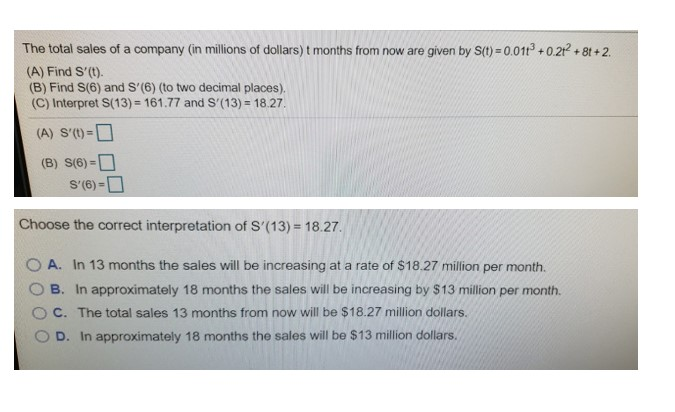# The total sales of a company (in millions of dollars) t months from now are given by S(1) = 0.011° + 0.21 + 8t +2.(A) Find S'(t).(B) Find S(6) and S'(6) (to two decimal places).(C) Interpret S(13) = 161.77 and S'(13) = 18.27.(A) S'(t)=D(B) S(6) =OS'(6) =D%3DChoose the correct interpretation of S'(13) = 18.27.In 13 months the sales will be increasing at a rate of \$18.27 million per month.A.B. In approximately 18 months the sales will be increasing by \$13 million per month.C. The total sales 13 months from now will be \$18.27 million dollars.In approximately 18 months the sales will be \$13 million dollars.D.

Question
10 viewshelp_outlineImage TranscriptioncloseThe total sales of a company (in millions of dollars) t months from now are given by S(1) = 0.011° + 0.21 + 8t +2. (A) Find S'(t). (B) Find S(6) and S'(6) (to two decimal places). (C) Interpret S(13) = 161.77 and S'(13) = 18.27. (A) S'(t)=D (B) S(6) =O S'(6) =D %3D Choose the correct interpretation of S'(13) = 18.27. In 13 months the sales will be increasing at a rate of \$18.27 million per month. A. B. In approximately 18 months the sales will be increasing by \$13 million per month. C. The total sales 13 months from now will be \$18.27 million dollars. In approximately 18 months the sales will be \$13 million dollars. D. fullscreen
check_circle

Step 1

According to the given information, the total sale of a company t months from now are given by:

Step 2

For part (A) it is required to calculate S’(t):

Step 3

Now, it is required to calculat...

### Want to see the full answer?

See Solution

#### Want to see this answer and more?

Solutions are written by subject experts who are available 24/7. Questions are typically answered within 1 hour.*

See Solution
*Response times may vary by subject and question.
Tagged in
MathCalculus

### Derivative